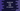# 2 different JavaScript program to calculate age from date of birth### Introduction:

In this post, I will show you how to find the age of a person based on the birthdate. i.e. we will take the year, month and day of birth as input and print out the age.

We will write one JavaScript program that will :

• take the user birthdate as input
• Prints out the age.

### JavaScript program:

Let’s take a look at the below program:

``````const userDOB = new Date("1989/11/17");
const today = new Date();

const msDiff = today - userDOB;
const age = Math.floor(msDiff / (365.25*24*60*60*1000))

console.log(age)``````

If today is Nov, 17, 2020, it will print 31.

• Here, userDOB is the Date object of user date of birth.
• today is the Date object for current date.
• msDiff variable holds the difference of today and userDOB. This difference is in milliseconds.
• We are diving with the total milliseconds in a year to find out the age for the user whose date of birth is given.

Here,

365.25 - Total days in a year 24 - Hours in one day 60 - Mins in one hour 60 - seconds in one minute 1000 - Converting the second value to milliseconds.

### Using moment.js :

moment.js is a popular javascript library used for date/time handling. You can check their homepage to learn more on this and how to add it to your project.

Now, let me show you how it will look like if I use momentjs :

``````const userDOB = moment('1989/11/17', 'YYYY/M/D');

const age = moment().diff(userDOB, 'years')

console.log(age)``````

Just one line of code to get the years difference. You can also find the difference in months, days etc.

moment.js is a lightweight library and I prefer to use it than the previous option.